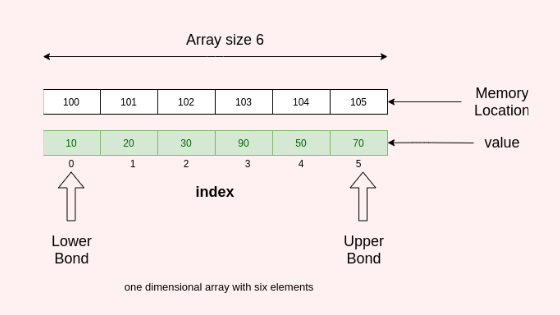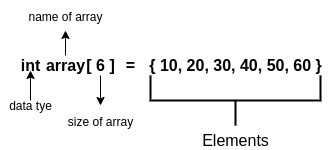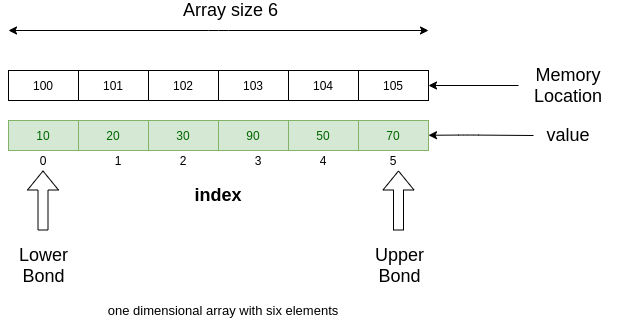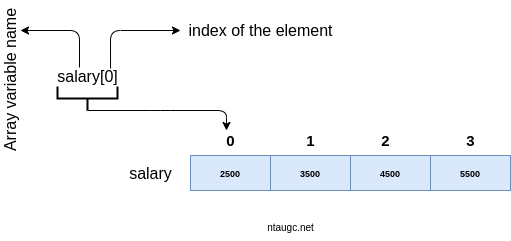# Array# Array

.

## What is an Array?

An array is a collection of similar data types that store items in contiguous memory locations. It is also known as the Subscripted variable.

Arrays are very powerful data structures that store lists of elements

Arrays are classified as Homogeneous Data Structures because they store elements of the same type. They can store numbers, strings, boolean values (true and false), characters, objects, and so on.

But once you define the type of values that your array will store, all its elements must be of that same type. You can’t mix different types of data.

For example, an int data type array stores int type elements while a char array contains char type elements. Following are the important terminology to understand the concept of Array.

• Index − Each element  is stored in a specific numerical location this is known as index.

• Element – Each item stored in an array or subscripted variable is known as an element.
• zero-based indexing  −  The first element  is indexed by the subscript of 0

• one-based indexing − The first element  is indexed by the subscript of 1

• subscript – index number is also known as a subscript.

## Why we need an Array

Suppose you want to store the salaries of 300 employees in ascending order. In such cases, we have two options for storing these salaries. One is an individual variable other is array or Subscripted variable.

1. Construct 300 variables to store the salaries of 300 employees, that is, each variable will contain the salary of one employee.
2. Construct a variable capable of storing or holding all three hundred values.

Of course, the second alternative is better. A simple reason for this is, it would be much easier to handle one variable than handling 300 different variables.

## Array Initialization and Representation

In this section, we know The statement used to declear an array is.

###### Initialization##### RepresentationAs per the above example, the following important points have to be considered.

• Index starts with 0 which is known as a lower bond.
• Last element index is known as upper bond
• Its length is six which means it can store 6 elements.
• Each element can be accessed via its index as shown below.##### Basic Operations

Following are the basic operations which is supported.

• Traverse − print all the elements one by one.

• InsertionIn the given index, adds an element.

• DeletionDeletes an item in the indicated index.

• Search – Searches for an element using the index given or by the value.

• UpdateIn the given index, updates an element.

###### Defaults values

Data Type Default Value
int0
char0
float0.0
boolfalse
void
double0.0f

In the case of a single-dimension Subscripted variable

total bytes = sizeof(base type) X size of array

For a two-dimensional Subscripted variable

total bytes = size of 1st index X size of 2nd index X sizeof(base type)

## Time complexity of various tasks or operation

OperationAverage CaseWorst Case
InsertO(n)O(n)
DeleteO(n)O(n)
SearchO(n)O(n)

• subscripted variable allows random access of elements.
• Subscripted variable has better cache locality so iterating the arrays using their index is faster compared to any others.
• It is multidimensional. 2D represents the matrix.
• An array is quite simple to implement other than data structures.